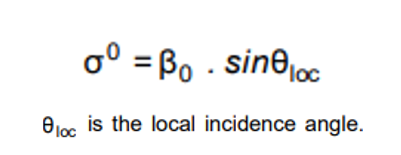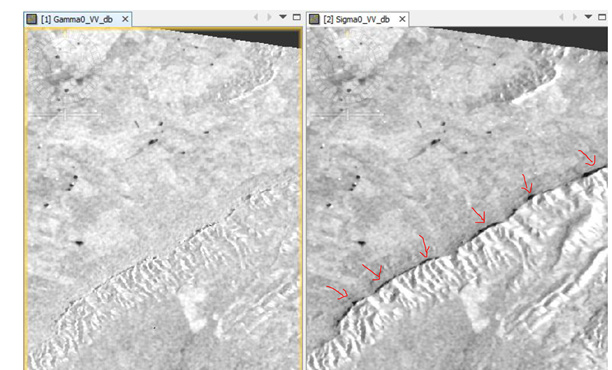# Help with Clarifying Radiometric Normalization of Full Scene Wide Swath Incidence Angle Effects

This topic has been well covered by this forum, but I would really appreciate some clarification and verification of my current thoughts (apologies for the length).

I have been trying to radiometrically normalize the effects of incidence angle on width swath IW Sentinel-1. The goal would be to determine what are the effects on crop returns in the near range compared to the far range. I will try to be as clear as possible what I mean by this, and what tools in SNAP I have tried to use. This would be the radiometric normalization of the changing incidence angle across the field of view of the sensor in the range direction. This is different from the radiometric normalization of terrain effects utilizing local incidence angle derived from the SRTM DEM. I realize now that these normalizations are different (the realization that even though you normalize using local incidence angle, IN GENERAL the local incidence angles used in the normalization will be smaller in the near range and IN GENERAL will be larger in the far range). I was missing this earlier and it caused me some confusion.

Given that, starting with using the Range Doppler Terrain Correction tool, selecting Radiometric Normalization option, this tool should take the backscatter in Sigma0 or Intensity and do a correction for local terrain effects on incidence angle using the equation in the help:If you input Intensity, the tool will create a Sigma0, and depending on the option in the Radiometric Normalization, use local incidence angle from DEM, projected local incidence angle from DEM, or incidence angle from ellipsoid. The tool presumably will create a virtual band that has that math equation defining the correction using the DEM local incidence angle, the ellipsoid incidence angle, and the Ellipsoid based Sigma0 backscatter input. My intuition tells me that the Sigma0 band created by this tool should be the same as created by the Calibration tool. This doesn’t seem to be the case. I can’t explain why. In addition, the equation that defines the local incidence angle normalized virtual band does not match the equation from the help (I am not great with math so I could be wrong about this). Finally, it seems to me the 2 options that use local incidence angle and projected local incidence angle never use the ellipsoid incidence angle, which is the whole point of the correction (or so I think). The results of using projected local incidence angle have 2 Sigma0 bands, no incidence angles, and no virtual bands. The results of using local incidence angle and ellipsoid incidence angle produce Sigma0, incidence angles, and virtual bands describing the normalization correction. So my question is: what exactly is the Range Doppler tool doing, in that I have such a hard time understanding going from a simple equation that relates ellipsoid incidence angle, DEM local incidence angle, and Sigma0 backscatter, to the actual results that the Range Doppler tool outputs. So I need help understanding the outputs of local terrain correction tool, but that isn’t my main issue as I have illustrated to begin with (more of a side problem once I realized the difference between local and overall range normalization). The different outputs from range doppler when using radiometric normalization, along with the virtual band equations are shown below. Wouldn’t local terrain radiometric normalization always need ellipsoid angle and local DEM angle?

From top to bottom, input product, RD_TC with projected local DEM, RD_TC with local DEM, RD_TC with ellipsoid. Note the equations for the 2 with virtual bands.

To do over range radiometric correction, you need to model the relationship between the target surface characteristics and the incidence angle of the incoming radiation, so that a proper compensation factor can be applied (different targets respond differently at varying incidence angles). What clued me in to this was this paper:

Section 3.8 on local terrain correction normalization, and Section 3.9 on over range incidence angle normalization. Here the author presents a cosine correction with a default factor of 2.

That factor would change depending on the model between target and incidence angle interaction. So, do tools exist in SNAP to perform this kind of over range normalization, even in a naive way using the same weighting factor for the entire scene. Would using the approach in the paper by using band math to manually do all the equations be a reasonable way to accomplish this? Is there something I’m missing or is the approach presented in the paper even the right way to accomplish this task?

In summary my questions are:

Am I thinking about the difference between over range incidence angle rad. correction and local terrain incidence angle rad. correction?

What are the outputs from the Range Doppler Terrain Correction (with radiometric norm option), tool, why does Sigma0 output from it not equal the Sigma0 output from Calibration. Why do some options for radiometric norm output virtual bands (local dem, ellipsoid) and some don’t (projected local dem)? Why do the equations in the virtual bands not match with the equation in the help? Why do the equations not always involved the ellipsoid angle, as that is the thing we are correcting from to get to local terrain angle?

Can SNAP perform over range incidence angle radiometric normalization? What is the best way to accomplish this if not?

Sorry for the post, but I thought it best to be clear as possible as the term radiometric normalization gets used a lot in different contexts. I have questions about when Speckle Filtering should be done but I will leave that to another (much shorter) post!

1 Like

In SNAP the best correction you can do is the Radiometric Terrain Flattening, which takes care of artefacts caused by the terrain and incidence angle; It is based on the method published developed by @eyeinsky

David Small, "Flattening Gamma: Radiometric Terrain Correction for SAR imagery", IEEE Transaction on Geoscience and Remote Sensing, Vol. 48, No. 8, August 2011

Thanks for your reply. I’m still having a hard time determining how the Range Doppler tool is working. I’ve looked at the source code and I can’t determine where local incidence angle is used in the applyCalibration function for Sentinel1. I’m having trouble determining what the virtual band equations in createSigmaNoughtVirtualBand function mean. I suspect it has to do with the incidence angle encoded in the LUTs for each of Sigma, Beta, and Gamma nought (detailed here: https://sentinel.esa.int/documents/247904/685163/S1-Radiometric-Calibration-V1.0.pdf)

I would really like to understand fully whats going on so that I can properly normalize the backscatter values. I hope my problems make sense.

I do not think the local incidence angle is used in the calibration-operator. You should use the already implemented operators for calibration, thermal noise removal and radiometric terrain flattening in order to normalise the backscatter values.

I understand that Terrain Flattening tool will remove effects of local terrain, with Beta0 as input, and Gamma0 as output. Would using the Radiometric Normalization option in the Range Doppler Terrain Correction tool provide similar results for the Sigma0 domain?

The reason why I need to be sure of what each tool is outputting is I am trying to do analysis of effects of normalization (local terrain and over range) on crop classifications on full scene width S1 products. Essentially, I am trying to start at first principles for SAR processing, implementing equations for Radiometric Calibration and Normalization so I can be sure the outputs are what I expect. Right now, outputs from the Terrain Correction tool, for example, I am not sure conform with the equations presented by Kellendorf, for example.

I appreciate your help, but I hope I have explained why just applying the tools is not sufficient for my current situation.

The should bring the same results, this option is a remnant from older versions, I compared it once here: S1 radiometric correction

1 Like

Sorry I just discovered this discussion now. The local incidence angle is not the best source of information for flattening backscatter, for reasons laid out in my paper referenced by Marcus above. The incidence angle that is encoded in the LUTSs is from an ellipsoid-based model.

The angle was only ever introduced as a proxy for the local area, and since we can calculate the local area directly using the DEM, we should use that instead. That is what is done when one applies radiometric terrain flattening.

1 Like

Dear @mengdahl,

I’m working with S1_GRD_IW and I’m trying to understand how the Calibration operator (GPT) works. I’m a bit confused regarding the role of the Local Indicence Angle/Incidence Angle in the calculation of Sigma0.

When you say:

“I do not think the local incidence angle is used in the calibration-operator”.

you mean that the formula for the calculation of Sigma0 (below)source

should be replaced (for SNAP) with something similar:I apologize in case it doesn’t have any sense!

BR

Agreed: In my papers, I usually annotate the theta above with an “E” subscript to make it unambiguous that the angle is based on an ellipsoidal Earth model.

Terrain-flattened gamma nought is generated by correcting for DEM-induced radar geometry effects. After those corrections, the gamma nought can be subscripted with a T to unambiguously indicate that terrain corrections were applied to the image’s radiometry.

1 Like

Dear Professor Small @eyeinsky ,

I thank you for your innovative research work.

I’d like to take the opportunity to ask you a question.

I’m working (for my thesis) on developing an automatic flood detection procedure. I have decided to adopt the terrain flattened gamma0 (rather than sigma0) because, in my understanding, it reduces the probability to extract erroneous flooded areas as the back slope areas:Do you think that makes sense?

BR

I am a senior research scientist, not a Professor.

Without knowing the region and its geography it is difficult to be conclusive, but I agree that using flattened backscatter imagery, backslopes on mountains and hills should not have as low backscatter as in uncorrected (e.g. sigma0) data.